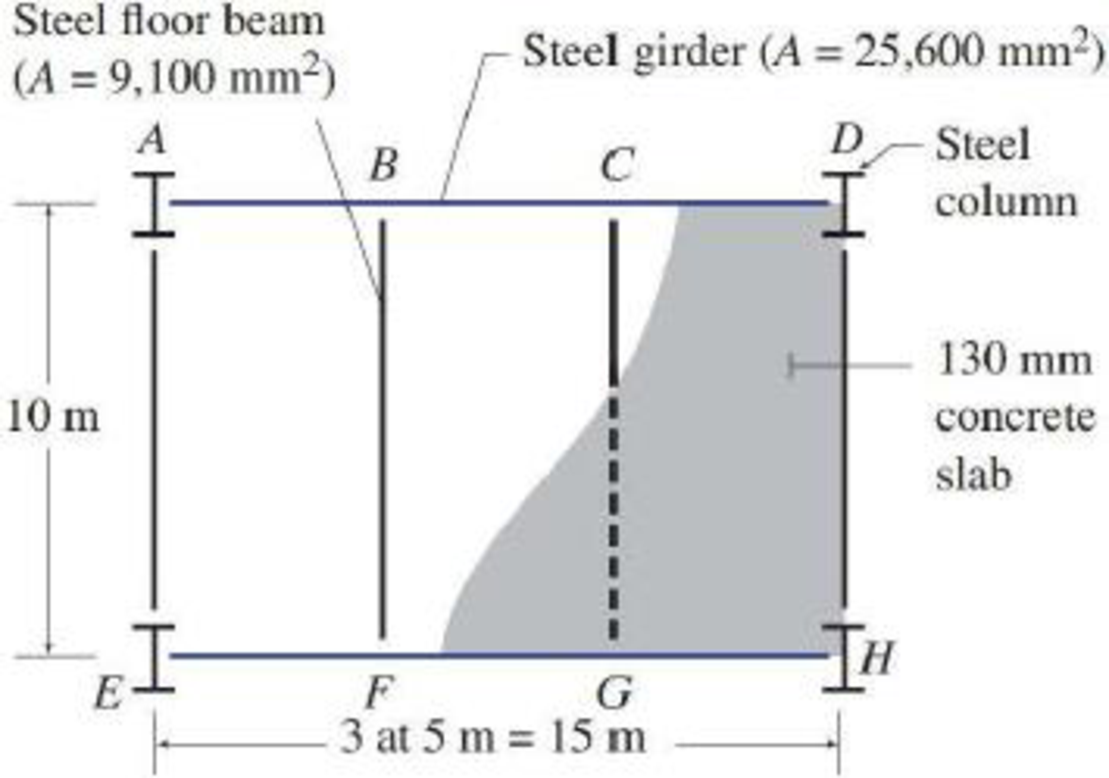# The floor system of a gymnasium consists of a 130 mm thick concrete slab resting on four steel beams ( A = 9,100 mm 2 ) that, in turn, are supported by two steel girders ( A = 25,600 mm 2 ), as shown in Fig. P2.7. Determine the dead loads acting on beam BF and girder AD . FIG. P2.7, P2.10

#### Solutions

Chapter
Section
Chapter 2, Problem 7P
Textbook Problem
1658 views

## The floor system of a gymnasium consists of a 130 mm thick concrete slab resting on four steel beams (A = 9,100 mm2) that, in turn, are supported by two steel girders (A = 25,600 mm2), as shown in Fig. P2.7. Determine the dead loads acting on beam BF and girder AD.FIG. P2.7, P2.10To determine

### Explanation of Solution

Given information:

Consider the floor system of gymnasium.

The thickness of the concrete slab is 130mm.

The area of the steel beams is Asb=9,100mm2.

The area of the steel girder is Asg=25,600mm2.

Calculation:

Show the slab as shown in Figure 1.

Refer to Figure 1.

Refer Table 2.1, “Unit Weight of the Construction Materials” in the textbook.

The unit of the structural steel is γs=77kN/m3.

The unit of the reinforced concrete is γc=23.6kN/m3.

Show the tributary area of the beam BF as shown in Figure 2.

Refer Figure 1 and Figure 2.

Calculate the dead load of concrete slab acting on  the beam per unit length of the beam as follows:

Calculate the dead load of steel beam acting per unit length of the beam as follows:

Dead of steel beam=γs×(UnitLengthoftheTributaryArea×Area of the steel beam)=77kN/m3×(1m×9100mm2×(1m1000mm)2)=77×(1×9100×106)0.7007kN

Calculate the total load acting on the steel beam per unit length of the beam as follows:

The total dead load is distributed uniformly over the entire length of the beam

### Still sussing out bartleby?

Check out a sample textbook solution.

See a sample solution

#### The Solution to Your Study Problems

Bartleby provides explanations to thousands of textbook problems written by our experts, many with advanced degrees!

Get Started

Find more solutions based on key concepts
For Example 3 in Table 18.8, verify that the given solution satisfies the governing differential equation and t...

Engineering Fundamentals: An Introduction to Engineering (MindTap Course List)

Write a query to count the number of customers with a balance of more than 500.

Database Systems: Design, Implementation, & Management

What are the three main task patterns? Provide an example of each.

Systems Analysis and Design (Shelly Cashman Series) (MindTap Course List)

Explain the process of product activation.

Enhanced Discovering Computers 2017 (Shelly Cashman Series) (MindTap Course List)

Given that P=120lb and Q=130lb, find the rectangular representation of P+Q.

International Edition---engineering Mechanics: Statics, 4th Edition

What is the maximum width of the cover pass? Why?

Welding: Principles and Applications (MindTap Course List)

If your motherboard supports ECC DDR3 memory, can you substitute non-ECC DDR3 memory?

A+ Guide to Hardware (Standalone Book) (MindTap Course List)